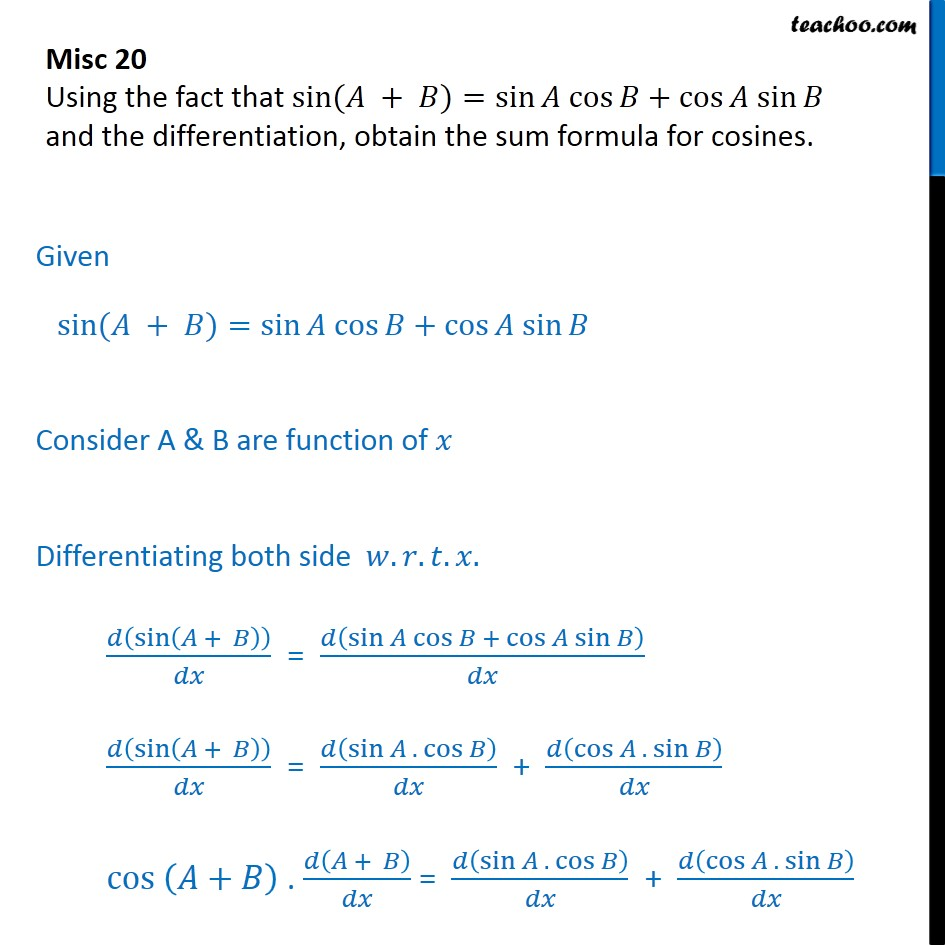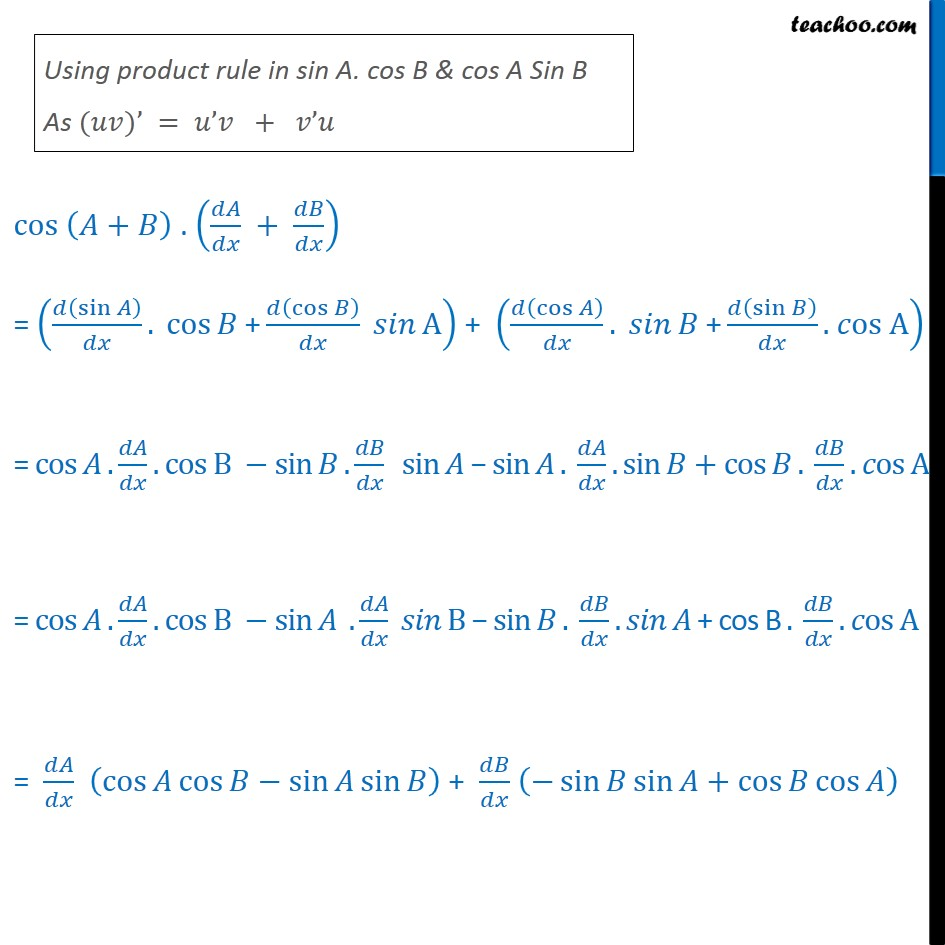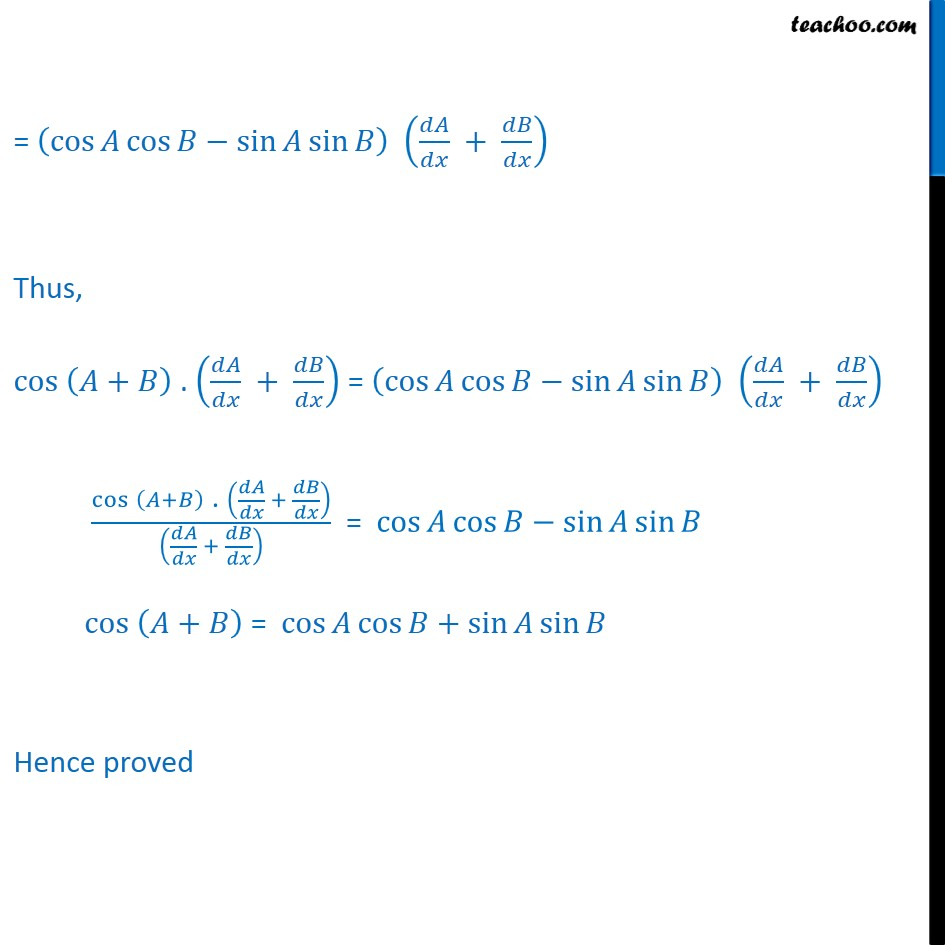Check Full Chapter Explained - Continuity and Differentiability - https://you.tube/Chapter-5-Class-12-Continuity1. Chapter 5 Class 12 Continuity and Differentiability
2. Serial order wise
3. Miscellaneous

Transcript

Misc 20 Using the fact that sin ( + )=sin cos +cos sin and the differentiation, obtain the sum formula for cosines. Given sin ( + )=sin cos +cos sin Consider A & B are function of Differentiating both side . . . . sin + = sin cos + cos sin sin + = sin . cos + cos . sin cos + . + = sin . cos + cos . sin cos + . + = sin . cos + cos A + cos . + sin . os A = cos . . cos B sin . sin sin . . sin + cos . . os A = cos . . cos B sin . B sin . . + cos B . . os A = cos cos sin sin + sin sin + cos cos = cos cos sin sin + Thus, cos + . + = cos cos sin sin + cos + . + + = cos cos sin sin cos + = cos cos + sin sin Hence proved

Miscellaneous